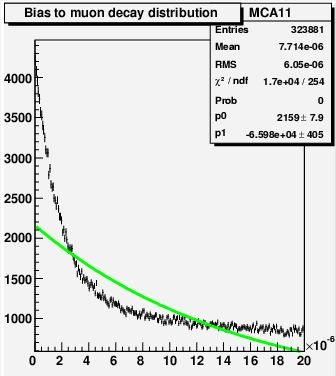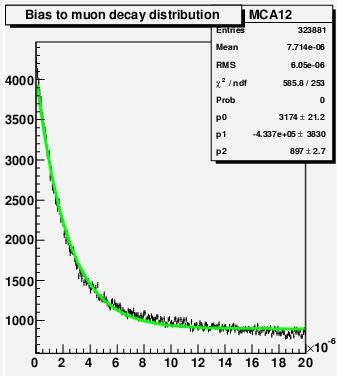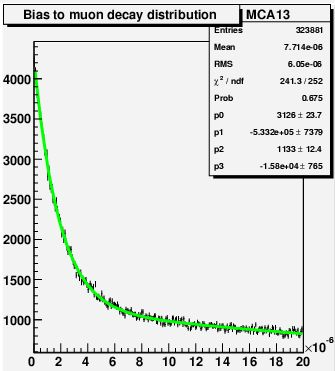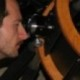## Answer to the muon decay experiment question June 5, 2008

Posted by dorigo in personal, physics, science.
Tags: , , , ,

Ok, one day has passed, and a few readers have decided to leave a comment with their answer to the question I posed in the former post, along with some additional considerations. I will first show the answer to the question pictorially, by pasting here four graphs I obtained this evening with a simple root macro. The macro perform a simple simulation of the experiment and -save some (unlikely) mistake on my part, it allows to obtain a histogram of counts in the 256 bins of a simulated multichannel analyzer (MCA), mapping the time frame$0 - 20 \mu s$ after a start has been detected by the experimental setup. The macro also allows the user to vary the number of bins of the MCA, the fraction of background, the exposure -but we need not be concerned with that.

A word of warning: the simulation is crude – I did not bother to insert the geometry of the apparatus (which may change from one setup to another), the energy loss of muons and electrons in Aluminum, or other details: I just simulated at random muon decays and background, recording whichever produces a stop in the MCA counts. Also, note that only positive muons are simulated here: as I warned in the post-scriptum to my post of yesterday, and as is further discussed in the thread to that post, negative muons get captured by Aluminum nuclei in the K-shell, and they decay much faster than positive ones -about 2.5 times more so. The decay produces a nuclear recoil which gets recorded in the scintillator as if it were an electron, causing in the end the MCA to record not one, but two different exponential distributions (with time constants of 2.2 and 0.88 microseconds for positive and negative muons, respectively) on top of random backgrounds. I ask the reader to forget about this detail in the following.In the plots you see the stop time distribution resulting from a million starts, in a situation when there is a large amount of background, and when a realistic fraction of positrons get detected by the apparatus (roughly 30%). The first plot shows the MCA distribution fit with a single exponential (answer #1). This is a very wrong modeling of what is happening, of course: in the presence of background -caused by particles randomly crossing scintillators B or C during the 20 microseconds of exposure after a real muon start- the multichannel will record both a muon decay signal with a time structure, and casual hits at random times.

The second plot shows how the data is interpreted with a functional form representing answer #2: a falling exponential plus a constant. Here things look definitely better, but a keen eye will spot the problem in the fit: the green curve stays above, then below, and then above the data points as we move left to right. In fact, in the upper right corner root informs us that the fit probability is zero! There is thus something wrong with answer #2.The third plot shows how the data is interpreted with a functional form representing answers #3 and #4: two falling exponentials with different time constants. The fit now is extremely good! The fit probability is a whooping 67.5%, indicating that our model of the data is correct, within the statistical power of the analyzed data. What makes options #3 and #4 different is that in the former case one expects one of the two exponentials to have a decay constant equal to the muon lifetime -which in the simulation was set to 2.0 microseconds for simplicity, while option #4 predicts that the lifetime measurement will be biased. Well, by looking at the parameters of the fitting function (which is$p_0 e^{p_1 t} + p_2 e^{p_3 t}$) one observes that neither$p_1$ nor$p_3$ have a value close to$-1/0.000002=-500,000$ (remember, the decay law is$e^{-t/\tau_\mu}$ so the fit parameter describing the exponential slope has to be the inverse of the opposite of the muon lifetime).

The fourth plot -at this point, only academic- shows the results of a constant fit. Of course, there is a lot of time dependence in the data, and not just a flat distribution!Now let me comment the result. Why are we finding that the background -which is uncorrelated with the time of arrival of stopped muons- shows a time structure ? And why on earth is the muon lifetime coming out biased low (the fit in the third plot has a value$p_0$ larger than 500,000) ?

The answer is not quite simple, but one can arrive at it by intuition, as I mentioned in the former post. First of all, we have to note that while the muon causing the start of our clock has one and only one chance to yield a signal -with the exponential law we discussed above-, backgrounds, however small, have multiple, albeit tiny, chances of providing counts in the time window: only the first arriving background count stops our clock and records an entry in the MCA, though. So, imagine you have not just one (and in our experimental setup, there is only a 20% chance that a background hit occurs in the 20 microseconds), but many background hits distributed at random throughout your 20 microseconds time window: they are overall flat -no time information from them- and yet, our data acquisition system records the first one! No wonder we get an exponential for the background as well!

So, the background produces an exponentially falling distribution, as showed by the fit in picture three. But why, however, do we get a wrong lifetime from the hits really coming from muon decays ?

The reason is the same as above. Muons decaying early on have a smaller chance of being beaten on time by a random background hit than muons decaying later on. So, the time distribution of real muon decay stops is also biased towards smaller values. We get a time constant smaller than the muon decay lifetime, that is.

Not convinced ? I can provide the root macro, and (by downloading the root package from cern) you should be able to test the system by yourself in minutes. Drop me a line if you wish to.1. Luboš Motl - June 6, 2008

Right, so one should better avoid guesses and solve it analytically.

There is now a lot of wrong things identified with the bad guesses above.

For example, the “buffer” may include more waiting muons than just one – in average – which is one of the reasons why they “stop the clock faster and why you’re getting a shorter lifetime.

To get the analytic result, let us first neglect the weak interactions in which the muon disappears. You need the following pre-requisite: the probability distribution “Q_N” that the buffer contains “N” muons at the average “start” moment. Note that “Q_0” equals zero by my assumption that every “start” means that a muon was captured.

Now, we have to make “Q_N(t)” functions of time, too – because the number of muons in the buffer is changing with time after “start”, too. Here, “Q_0(t)” is no longer zero.

Then you solve a system of equations for “Q_N(t)” as well as “P_N(t)” – probabilities that exactly “N” beeps have occurred around time “t” after the “start” moment. Here, “N” is any non-negative integer. Wherever Q_{-1} or P_{-1} appears in the equations, it should be erased:

dQ_N/dt = Q_{N+1} DecayRate + Q_{N-1} Inflying Rate
dP_N/dt = P_{N-1} (BgRate + SumOverN N . Q_N)

Here, the BgRate only contains the muons that penetrated while InFlying only contains the rate of muons that are being captured by the buffer.

The answer observed in the graphs and Tommaso’s question is P_1(t). The initial conditions for P are clearly P_0(0)=1, otherwise P_N(0)=0. The initial conditions for Q are calculated as the fixed point of the Q-equations above themselves because they are unaffected by P. 😉

Now fix a few errors in the equations if there are any and solve them. 😉2. Luboš Motl - June 6, 2008

The second term in the equation for P_N should have an extra factor of -DecayRate times P_N or something like that. 😉3. rockno3 - June 6, 20084. dorigo - June 6, 2008

Hi Lubos,

some perspective: a typical apparatus (say a half square meter bar of aluminum) will count roughly 10 muon stops per second. So the chance that a 20 microsecond window contains a second muon stop is one in 50,000. Three muons is one in 2.5 billion…

Also, differential calculus is not something I wish to explain to my audience. The exercise is useful because it forces people to think at the outcome of a gedankenexperiment; by doing so, an experimenter is able to design the apparatus in a way which minimizes the systematical uncertainties and increases the precision of the measurement. However, if you say it is trivial to compute the functional form, I encourage you to do so. I predict you will find two exponentials, with decay constants depending on the fraction of background, and neither of them equal to the muon lifetime (one of them a bit shorter, in fact).

Cheers,
T.5. Luboš Motl - June 6, 2008

Dear Tommaso, I believe that the two exponentials can be the correct answer if you neglect all lost muon processes and multiple muons in the buffer (1/50,000).

With these approximations, it should be trivial to solve it analytically and it is plausible that the solution is as easy as yours. Let me have a look.6. Luboš Motl - June 6, 2008

The decay rate of one muon is DecayRate.

The rate of muons that are creating fake stops (not counting the right ones) is NoiseRate.

So I believe that in your approximations, the probability P_0(t) that no signal occurred after time “t” is simply

P_0(t) = exp(-(NoiseRate+DecayRate)t).

The probability that there was one stop, P_1(t), satisfies

dP_1(t)/dt = P_0(t) (NoiseRate+DecayRate) – P_1(t) NoiseRate

The last term is the probability that you beep “stop” for the second time, which is only by noise by your assumption.

If the positive term proportional to P_0 on the RHS is neglected, the equation is solved as

P_1(t) = C(t) exp(-NoiseRate . t)

except that I allow C(t) to be non-constant, to account for the first positive term. We have for C:

dC/dt = exp(-DecayRate t) (DecayRate+NoiseRate)
C = -(DecayRate+NoiseRate)/DecayRate . exp(-DecayRate . t) + K

Now K is a real constant. It must be chosen so that for t=0, C(t) = 0, so that simply

C = (DecayRate+NoiseRate)/DecayRate . (1 – exp(-DecayRate . t))

and recall that

P_1(t) = C(t) exp(-NoiseRate . t)

So P_1(t) is a combination of two exponentials. The exponents are -t multiplied by NoiseRate (the positive coefficient) and NoiseRate+DecayRate (the negative coefficient), respectively.

The histogram is encoded in the time-derivative of P_1(t) which switches the sign of the coefficients. So the histogram is a positive coefficient times the dropping exponential of -t times NoiseRate+DecayRate plus a negative coefficient times the dropping exponential of -t times NoiseRate.

And you are proven preciously correct.

Best
Lubos7. dorigo - June 6, 2008

Hi Lubos,

I am home now and cannot put CPU into your equations -will do that next Monday (weekends are for my kids). However, even without checking the math, I can say I am happy you confirmed my interpretation. Thank you for carrying out the exercise.

Cheers,
T.8. Nicola - June 9, 2008

Hi,
why don’t shift the B paddle to the bottom of your apparatus?
With this setup (A on the top, BC on the bottom) using a trigger logic like
Start= A * not(B)*not(C)
Stop= not(A)*B*C
the false Starts are suppose to increase, but it should remove the bias due to false Stops, shouldn’t it?9. dorigo - June 9, 2008

Hi Nicola,

no, for two reasons.

One: you need to ensure that start signals are as signal-pure as possible. Now, going from (1) A*B*(notC) to (2) A*(notB)*(notC) as far as background rate is concerned is suicidal.

A typical counter will have some hundreds of counts per second. Say 100 Hz to be conservative. These are fast pulses which can be shaped into 50 ns-wide, 1.2V high square signals (if I remember correctly the voltage, but it is inessential). Now, with these background rates, random starts in configuration (2) above have a rate of 100Hz*(1-100Hz*50ns)*(1-100Hz*50ns)=99.999 Hz, while in configuration (1) they are 100Hz*(100Hz*50ns)*(1-100Hz*50ns)=4.999E-4 Hz. So in choosing your configuration increases random background counts by a factor 200,000 . Under those conditions you cannot measure anything any longer.

There is one further reason for avoiding that configuration in the way you suggest using. The decay positrons, as well as the recoil nuclei in case of muon k-capture (see comments above) have a hard time making it through the aluminum into the counter. Adding a second counter sizable decreases the rate of detectable decay signals.

False stops are indeed suppressed with a coincidence too, but the best way to address this is by either keeping the PMT voltages low (such that their efficiency is lower but their dark noise is reduced), or-if one has enough money- put two PMT tubes at opposite ends of the scintillators B,C. This way a coincidence can be done with the same crossing of an electron, and you get the advantage you mention without the problem of a decreased efficiency.

Cheers,
T.10. Arun - June 10, 2008

Trying simple arithmetic in a simple model:
e.g., 3/4 of the muons deterministically decay at time T and the remaining 1/4 at time 2T; the background arrives at T/2, T, 3T/2, 2T at a uniform rate B.

Then we get muon decay signals
at T/2 with a rate B = 3/4 B {spurious signal for muons that decay at T} + 1/4 B {spurious signal for muons that decay at 2T}
at T with a rate 3/4 – 3/4 B.
at 3T/2 with a rate 1/4 B
at 2T with a rate 1/4 – 1/4 B {background at T/2} – 1/4 B {background at 3T/2}

So we see the background appearing at {T/2, 3T/2} as {3/4B, 1/4B} – the background mimics the distribution of the muons.

The change in time constant is not so clearly visible here; but the decays appearing at T, 2T are 3/4 (1-B), 1/4 (1-2B) and the average decay time is 5/4 T – 7/4 B T instead of the correct 5/4 T.

Dunno if that is helpful to any readers.11. Arun - June 11, 2008

Correction to the above, assume background arrives if at all at T/2 and 3T/2 (and not at T or 2T).12. dorigo - June 11, 2008

Hi Arun,
sorry – I thought I had already answered your comment #10, now #11 reminded me I hadn’t.
I think it might be an intuitive way of handling the matter, were it not for the cumbersome math. In turn, let me propose my own “intuitive” solution for the background shape alone (forgetting signal, that is). If you have only one background stop in a 20 us window, it has a 50%-50% chance of happening in the first half or latter half of the time window. If there are two stops, they can both be in the first half (25% of the times), one in each (50% of the times) and both in the second (25% of the times): only in the latter 25% of cases will we get one hit in the second half. Now, if there are three hits, they distribute in 1/8, 3/8, 3/8, 1/8 fashion in the following ways: 111-0, 11-1, 1-11, 0-111. So only 12.5% of the times they generate one hit in the latter half.

If we sum these contributions, knowing that the rate of N hits goes with R^N (R<<1 Hz), we get the following series for hits recorded in the latter 10 us:

1/2 R + 1/4 R^2 + 1/8 R^3 + 1/16 R^4. ….

This is the fraction of hits. The total rate is

R + R^2 + R^3 + R^4 + …..

So, in the absence of signal, the background distributes in a way that has a higher number of total counts in the first half. How much higher ? well:$N_1 / N_2 = \frac{\Sigma_i R^i (2^i-1)/2^i}{ \Sigma_i R^i/2^i}$

Which is easy to compute but I have no time (following Fermat).

Cheers,
T.

13. Experimenter for one day - a subtlety in the muon lifetime measurement « A Quantum Diaries Survivor - September 5, 2008

[…] PPS: And the answer is here. […]

Sorry comments are closed for this entry